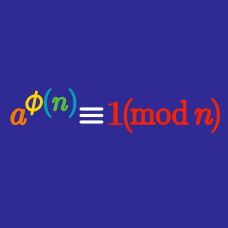Number Theory

# Euler's Theorem: Level 4 Challenges

$\large a^{11762}\equiv{a^2}\pmod{25725}$

Find the smallest positive integer $a$ such that the congruency above fails to hold.

What is the remainder when

$1^{2016}+2^{2016}+\cdots+2016^{2016}$

is divided by 2016?

A degree $5$ monic polynomial $P(x)$ has $5$ (not necessarily distinct) integer roots. Given that $P(0)=2014$, how many ordered quintuplets of roots $(r_1,r_2,r_3,r_4,r_5)$ satisfy the property that $r_1+r_2+r_3+r_4+r_5$ has the same units digit as $r_1^5+r_2^5+r_3^5+r_4^5+r_5^5$

Let $\phi(n)$ denote Euler's Totient Function. If the greatest common divisor of the positive integers $m$ and $n$ is 7, and $\phi(mn) = 5544,$ find the least possible value of $\left|\phi(m)-\phi(n)\right|$.

Suppose it takes $S$ digits in the binary expansion of $\frac{1}{{3}^{10}}$ for it to repeat. What is the minimum value of $S$?

As an example, $\frac{1}{3} = 0.01010101...$ in base two, which takes two digits to repeat.

×